# Fake Face Generator Using DCGAN ModelFake Face Generator Using DCGAN Model. In the following article, we will define and train a Deep Convolutional Generative Adversarial Network(DCGAN) model on a dataset of faces. The main objective of the model is to get a Generator Network to generate new images of fake human faces that look as realistic as possible.

## Overview

In the following article, we will define and train a Deep Convolutional Generative Adversarial Network_(DCGAN)_ model on a dataset of faces. The main objective of the model is to get a Generator Network to generate new images of fake human faces that look as realistic as possible.

To do so, we will first try to understand the intuition behind the working of GANs and DCGANs and then combine this knowledge to build a Fake Face Generator Model. By the end of this post, you will be able to generate your fake samples on any given dataset, using the concepts from this article.

## Introduction

The following article is divided into two sections:

• Theory — Understanding the intuition behind the working of _GAN_s and _DCGAN_s.
• Practical — Implementing Fake Face Generator in Pytorch.

This article would be covering both sections. So let’s begin the journey….

## Intuition Behind Generative Adversarial Networks(GANs)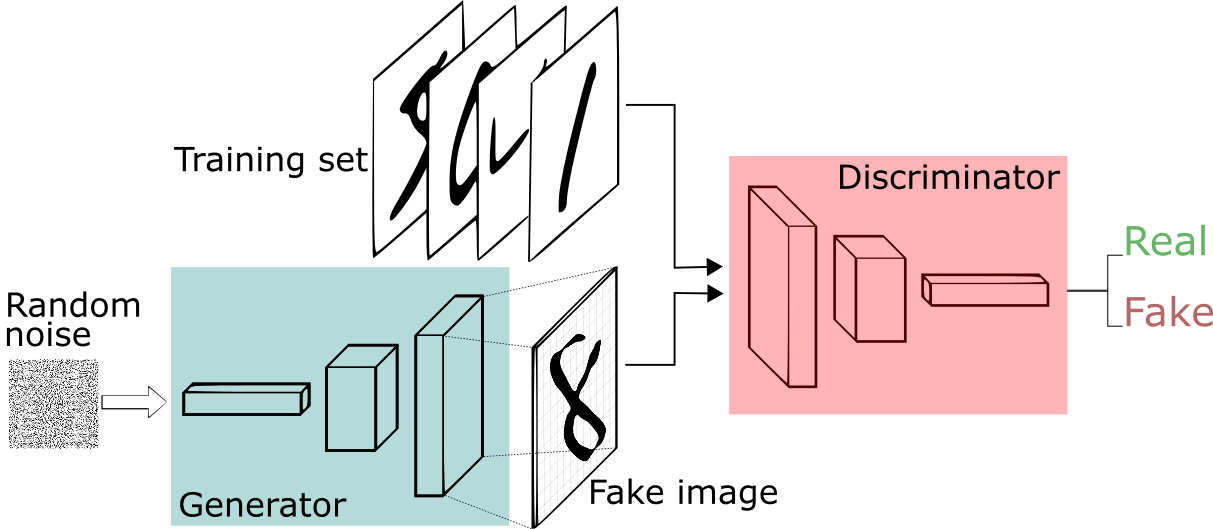• Definition

GAN_s, in general, can be defined as a generative model that lets us generate a whole image in parallel. Along with several other kinds of generative models, _GAN_s uses a differentiable function represented by a neural network as a _Generator Network.

• Generator Network

The Generator Network takes random noise as input, then runs the noise through the differentiable function_(neural network)_ to transform the noise and reshape it to have a recognizable structure similar to the images in the training dataset. The output of the Generator is determined by the choice of the input random noise. Running the Generator Network over several different random input noises results in different realistic output images.

The end goal of the Generator is to learn a distribution similar to the distribution of the training dataset to sample out realistic images. To be able to do so, the Generator Network needs to be trained. The training process of the GAN_s is very different, compared to other generative models( Most generative models are trained by adjusting the parameters to maximize the probability of Generator to generate realistic samples.For-eg Variational Auto-Encoders(VAE)). GAN_s, on the other hand, uses a second network to train the Generator, called Discriminator Network.

• Discriminator Network

Discriminator Network is a basic classifier network that outputs the probability of an image to be real. So, during the training process, the Discriminator Network is shown real images from the training set half the time and fake images from Generator another half of the time. The Discriminator target is to assign a probability near 1, for real images and probability near 0, for fake images.

On the other hand, Generator tries the opposite, its target is to generate fake images, for which Discriminator would result in a probability close to 1_(considering them to be real images from the training set)_. As training goes on, the Discriminator will become better at classifying real and fake images. So to fool the Discriminator, Generator will be forced to improve to produce more realistic samples. So we can say that:

GANs can be considered as a two-player(Generator and Discriminator)non-cooperative game, where each player wishes to minimize its cost function.

### Difference Between GANs and DCGANs

_DCGAN_s are very similar to _GAN_s but specifically focuses on using deep convolutional networks in place of fully-connected networks used in Vanilla _GAN_s.

Convolutional networks help in finding deep correlation within an image, that is they look for spatial correlation. This means DCGAN would be a better option for image/video data, whereas GAN_s can be considered as a general idea on which _DCGAN_s and many other architectures _(CGAN, CycleGAN, StarGAN and many others) have been developed.

In this article, we are mainly working on image data, this means that DCGAN would be a better option compared to Vanilla GAN. So from now on, we will be mainly focussing on DCGANs.

### Some Tips For Training DCGANs

All the training tips can also be applied toVanilla GAN_s_ as well_._

• Make sure that both Discriminator and Generator have at least one hidden layer. This makes sure both the models have a Universal Approximation Property.

Universal Approximation Propertystates that a feed-forward network with a single hidden layer containing a finite number of hidden units can approximate any probability distribution, given enough hidden units.

• For the hidden units, many activation functions could work, but Leaky ReLUs are the most popular. Leaky ReLUs make sure that gradient flows through the entire architecture. This is very important for _DCGAN_s because the only way the Generator can learn is to receive a gradient from the Discriminator.
• One of the most popular activation function for the output of the Generator Network is the Tangent Hyperbolic Activation Function_(Based on the_ Improved Training Techniques For GANs paper).
• As the Discriminator is a binary classifier, we will be using the Sigmoid Activation Function to get the final probability.

So far, we have talked about the working intuition and some tips and tricks for training _GAN_s/_DCGAN_s. But still, many questions are left unanswered. Some of them are:

Which optimizer to choose? How is the cost function defined? How long does a network need to be trained? and many others, that would be covered in the Practical section.

## Practical

The implementation part is broken down into a series of tasks from loading data to defining and training adversarial networks. At the end of this section, you’ll be able to visualize the results of your trained Generator to see how it performs; your generated samples should look fairly like realistic faces with small amounts of noise.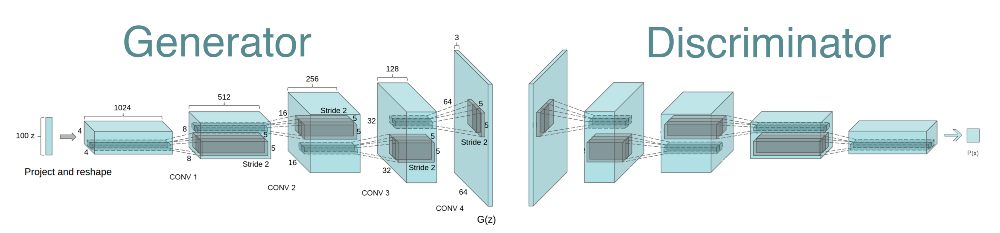### (1)Get The Data

You’ll be using the CelebFaces Attributes Dataset (CelebA) to train your adversarial networks. This data is a more complex dataset compared to the MNIST. So, we need to define a deeper network_(DCGAN)_ to generate good results. I would suggest you use a GPU for training purposes.

### (2)Preparing Data

As the main objective of this article is building a DCGAN model, so instead of doing the preprocessing ourselves, we will be using a pre-processed dataset. You can download the smaller subset of the CelebA dataset from here. And if you are interested in doing the pre-processing, do the following:

• Crop images to remove the part that doesn’t include the face.
• Resize them into 64x64x3 NumPy images.

Now, we will create a DataLoader to access the images in batches.

``````def get_dataloader(batch_size, image_size, data_dir='train/'):
"""
Batch the neural network data using DataLoader
:param batch_size: The size of each batch; the number of images in a batch
:param img_size: The square size of the image data (x, y)
:param data_dir: Directory where image data is located
"""
transform = transforms.Compose([transforms.Resize(image_size),transforms.CenterCrop(image_size),transforms.ToTensor()])

dataset = datasets.ImageFolder(data_dir,transform = transform)

batch_size = 256

• You can decide on any reasonable batch_size parameter.
• However, your image_size must be 32. Resizing the data to a smaller size will make for faster training, while still creating convincing images of faces.

Next, we will write some code to get a visual representation of the dataset.

``````def imshow(img):
npimg = img.numpy()
plt.imshow(np.transpose(npimg, (1, 2, 0)))# obtain one batch of training images
images, _ = dataiter.next() # _ for no labels# plot the images in the batch, along with the corresponding labels
fig = plt.figure(figsize=(20, 4))
plot_size=20
for idx in np.arange(plot_size):
ax = fig.add_subplot(2, plot_size/2, idx+1, xticks=[], yticks=[])
imshow(images[idx])``````

Keep in mind to convert the Tensor images into a NumPy type and transpose the dimensions to correctly display an image based on the above code_(In Dataloader we transformed the images to Tensor)_. Run this piece of code to get a visualization of the dataset.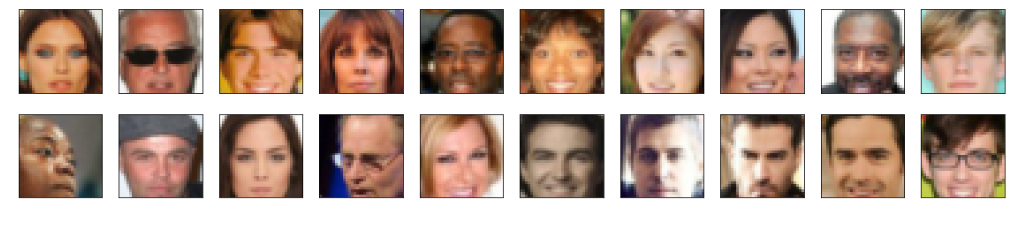Now before beginning with the next section_(Defining Model),_ we will write a function to scale the image data to a pixel range of -1 to 1 which we will use while training. The reason behind doing so is that the output of a tanh activated generator will contain pixel values in a range from -1 to 1, and so, we need to rescale our training images to a range of -1 to 1_(right now, they are in the range 0–1)_.

``````def scale(x, feature_range=(-1, 1)):
''' Scale takes in an image x and returns that image, scaled
with a feature_range of pixel values from -1 to 1.
This function assumes that the input x is already scaled from 0-1.'''
# assume x is scaled to (0, 1)
# scale to feature_range and return scaled x
min, max = feature_range
x = x*(max-min) + min
return x``````

## (3)Defining Model

A GAN is comprised of two adversarial networks, a discriminator and a generator. So in this section, we will define architectures for both of them.

### Discriminator

This is a convolutional classifier, only without any MaxpPooling layers. Here is the code for the Discriminator Network.

``````def conv(input_c,output,kernel_size,stride = 2,padding  = 1, batch_norm = True):
layers =[]
layers.append(con)

if batch_norm:
layers.append(nn.BatchNorm2d(output))

return nn.Sequential(*layers)class Discriminator(nn.Module):def __init__(self, conv_dim):
"""
Initialize the Discriminator Module
:param conv_dim: The depth of the first convolutional layer
"""
#complete init functionsuper(Discriminator, self).__init__()
self.conv_dim = conv_dim
self.layer_1 = conv(3,conv_dim,4,batch_norm = False) #16
self.layer_2 = conv(conv_dim,conv_dim*2,4) #8
self.layer_3 = conv(conv_dim*2,conv_dim*4,4) #4
self.fc = nn.Linear(conv_dim*4*4*4,1)def forward(self, x):
"""
Forward propagation of the neural network
:param x: The input to the neural network
:return: Discriminator logits; the output of the neural network
"""
# define feedforward behavior
x = F.leaky_relu(self.layer_1(x))
x = F.leaky_relu(self.layer_2(x))
x = F.leaky_relu(self.layer_3(x))
x = x.view(-1,self.conv_dim*4*4*4)
x = self.fc(x)
return x``````

Explanation

• The following architecture consists of three convolutional layers and a final fully connected layer, which output a single logit. This logit defines whether the image is real or not.
• Each convolution layer, except the first one, is followed by a Batch Normalization(defined in conv helper function).
• For the hidden units, we have used the leaky ReLU activation function as discussed in the theory section.
• After each convolution layer, the height and width become half. For-eg After the first convolution 32X32 images will be resized into 16X16 and so on.

Output dimension can be calculated using the following formula: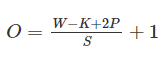where O is the output height/length, W is the input height/length, K is the filter size, P is the padding, and S is the stride.

• The number of feature maps after each convolution is based on the parameter conv_dim(In my implementation conv_dim = 64).

In this model definition, we haven’t applied the Sigmoid activation function on the final output logit. This is because of the choice of our loss function. Here instead of using the normal BCE(Binary Cross-Entropy Loss), we will be using BCEWithLogitLoss, which is considered as a numerically stable version of BCE. BCEWithLogitLoss is defined such that it first applies Sigmoid activation function on the logit and then calculates the loss, unlike BCE. You can read more about these loss functions here.

## Generator

The generator should upsample an input and generate a new image of the same size as our training data 32X32X3. For doing so we will be using transpose convolutional layers. Here is the code for the Generator Network.

``````def deconv(input_c,output,kernel_size,stride = 2, padding =1, batch_norm = True):
layers = []
layers.append(decon)

if batch_norm:
layers.append(nn.BatchNorm2d(output))
return nn.Sequential(*layers)class Generator(nn.Module):

def __init__(self, z_size, conv_dim):
"""
Initialize the Generator Module
:param z_size: The length of the input latent vector, z
:param conv_dim: The depth of the inputs to the *last* transpose convolutional layer
"""
super(Generator, self).__init__()
# complete init function
self.conv_dim = conv_dim
self.fc = nn.Linear(z_size,conv_dim*8*2*2)
self.layer_1 = deconv(conv_dim*8,conv_dim*4,4) #4
self.layer_2 = deconv(conv_dim*4,conv_dim*2,4) #8
self.layer_3 = deconv(conv_dim*2,conv_dim,4) #16
self.layer_4 = deconv(conv_dim,3,4,batch_norm = False) #32

def forward(self, x):
"""
Forward propagation of the neural network
:param x: The input to the neural network
:return: A 32x32x3 Tensor image as output
"""
# define feedforward behavior
x = self.fc(x)
x = x.view(-1,self.conv_dim*8,2,2) #(batch_size,depth,width,height)
x = F.relu(self.layer_1(x))
x = F.relu(self.layer_2(x))
x = F.relu(self.layer_3(x))
x = torch.tanh(self.layer_4(x))
return x``````

Explanation

• The following architecture consists of a fully connected layer followed by four transpose convolution layers. This architecture is defined such that the output after the fourth transpose convolution layer results in an image of dimension 32X32X3_(size of an image from training dataset)._
• The inputs to the generator are vectors of some length zsize(z_size is the noise vector)_.
• Each transpose convolution layer, except the last one, is followed by a Batch Normalization(defined in deconv helper function).
• For the hidden units, we have used the ReLU activation function.
• After each transpose convolution layer, the height and width become double. For-eg After the first transpose convolution 2X2 images will be resized into 4X4 and so on.

Can be calculated using the following formula:

`_# Padding==Same: H = H1 * stride_`

`_# Padding==Valid H = (H1-1) * stride + HF_`

where H = output size, H1 = input size, HF = filter size.

• The number of feature maps after each transpose convolution is based on the parameter conv_dim(In my implementation conv_dim = 64).

## (4)Initialize The Weights Of Your Networks

To help the models converge, I initialized the weights of the convolutional and linear layers in the model based on the original DCGAN paper, which says: All weights are initialized from a zero-centered Normal distribution with a standard deviation of 0.02.

``````def weights_init_normal(m):
"""
Applies initial weights to certain layers in a model .
The weights are taken from a normal distribution
with mean = 0, std dev = 0.02.
:param m: A module or layer in a network
"""
# classname will be something like:
# `Conv`, `BatchNorm2d`, `Linear`, etc.
classname = m.__class__.__name__

if hasattr(m,'weight') and (classname.find('Conv') != -1 or classname.find('Linear') != -1):

m.weight.data.normal_(0.0,0.02)

if hasattr(m,'bias') and m.bias is not None:
m.bias.data.zero_()``````
• This would initialize the weights to a normal distribution, centered around 0, with a standard deviation of 0.02.
• The bias terms, if they exist, maybe left alone or set to 0.

## (5)Build Complete Network

Define your models’ hyperparameters and instantiate the discriminator and generator from the classes defined in the Defining Model section. Here is the code for that.

``````def build_network(d_conv_dim, g_conv_dim, z_size):
# define discriminator and generator
D = Discriminator(d_conv_dim)
G = Generator(z_size=z_size, conv_dim=g_conv_dim)# initialize model weights
D.apply(weights_init_normal)
G.apply(weights_init_normal)print(D)
print()
print(G)

return D, G

# Define model hyperparams
d_conv_dim = 64
g_conv_dim = 64
z_size = 100D, G = build_network(d_conv_dim, g_conv_dim, z_size)``````

When you run the above code you get the following output. It also describes the model architecture for the Discriminator and Generator models.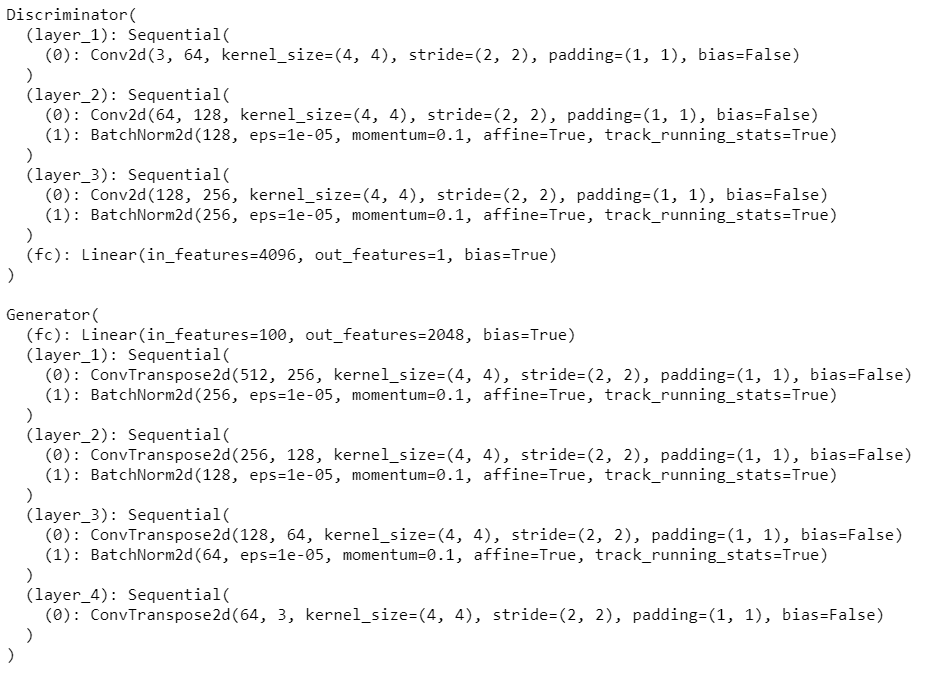## (6)Training Process

The training process comprises defining the loss functions, selecting optimizer and finally training the model.

## Discriminator And Generator Loss

Discriminator Loss

• For the discriminator, the total loss is the sum of (d_real_loss + d_fake_loss), where dreal_loss_ is the loss obtained on images from the training data and dfake_loss_ is the loss obtained on images generated from Generator Network. For-eg

z — Noise vector

i — Image from the training set

G(z) — Generated image

D(G(z)) — Discriminator output on a generated image

D(i) — Discriminator output on a training dataset image

Loss = real_loss(D(i)) + fake_loss(D(G(z)))

• Remember that we want the Discriminator to output 1 for real images and 0 for fake images, so we need to set up the losses to reflect that_(Keep this line in mind while reading the code below)_.

Generator Loss

• The Generator loss will look similar only with flipped labels. The generator’s goal is to get the discriminator to think its generated images are real. For-eg

z — Noise vector

G(z) — Generated Image

D(G(z)) — Discriminator output on a generated image

Loss = real_loss(D(G(z)))

Here is the code for real_loss and fake_loss

``````def real_loss(D_out):
'''Calculates how close discriminator outputs are to being real.
param, D_out: discriminator logits
return: real loss'''
batch_size = D_out.size(0)
labels = torch.ones(batch_size)
if train_on_gpu:
labels = labels.cuda()
criterion = nn.BCEWithLogitsLoss()
loss = criterion(D_out.squeeze(),labels)
return lossdef fake_loss(D_out):
'''Calculates how close discriminator outputs are to being fake.
param, D_out: discriminator logits
return: fake loss'''
batch_size = D_out.size(0)
labels = torch.zeros(batch_size)
if train_on_gpu:
labels = labels.cuda()
criterion =  nn.BCEWithLogitsLoss()
loss = criterion(D_out.squeeze(),labels)
return loss``````

### Optimizers

For GAN_s we define two optimizers, one for the Generator and another for the Discriminator. The idea is to run them simultaneously to keep improving both the networks. In this implementation, I have used [_Adam optimizer](https://arxiv.org/pdf/1412.6980.pdf) in both cases. To know more about different optimizers, refer to this link.

``````# Create optimizers for the discriminator D and generator G
d_optimizer = optim.Adam(D.parameters(),lr = .0002, betas = [0.5,0.999])
g_optimizer = optim.Adam(G.parameters(),lr = .0002, betas = [0.5,0.999])``````

Learning rate(lr) and betas values are based on the original DCGAN paper.

### Training

Training will involve alternating between training the discriminator and the generator. We’ll use the real_loss and fake_loss functions defined earlier, to help us in calculating the Discriminator and Generator losses.

• You should train the discriminator by alternating on real and fake images
• Then the generator, which tries to trick the discriminator and should have an opposing loss function

Here is the code for training.

``````def train(D, G, n_epochs, print_every=50):
'''Trains adversarial networks for some number of epochs
param, D: the discriminator network
param, G: the generator network
param, n_epochs: number of epochs to train for
param, print_every: when to print and record the models' losses
return: D and G losses'''

# move models to GPU
if train_on_gpu:
D.cuda()
G.cuda()# keep track of loss and generated, "fake" samples
samples = []
losses = []# Get some fixed data for sampling. These are images that are held
# constant throughout training, and allow us to inspect the model's performance
sample_size=16
fixed_z = np.random.uniform(-1, 1, size=(sample_size, z_size))
fixed_z = torch.from_numpy(fixed_z).float()
# move z to GPU if available
if train_on_gpu:
fixed_z = fixed_z.cuda()# epoch training loop
for epoch in range(n_epochs):# batch training loop
for batch_i, (real_images, _) in enumerate(celeba_train_loader):batch_size = real_images.size(0)
real_images = scale(real_images)
if train_on_gpu:
real_images = real_images.cuda()

# 1. Train the discriminator on real and fake ima.ges
d_out_real = D(real_images)
z = np.random.uniform(-1,1,size = (batch_size,z_size))
z = torch.from_numpy(z).float()
if train_on_gpu:
z = z.cuda()
d_loss = real_loss(d_out_real) + fake_loss(D(G(z)))
d_loss.backward()
d_optimizer.step()
# 2. Train the generator with an adversarial loss
G.train()
z = np.random.uniform(-1,1,size = (batch_size,z_size))
z = torch.from_numpy(z).float()
if train_on_gpu:
z = z.cuda()
g_loss = real_loss(D(G(z)))
g_loss.backward()
g_optimizer.step()

# Print some loss stats
if batch_i % print_every == 0:
# append discriminator loss and generator loss
losses.append((d_loss.item(), g_loss.item()))
# print discriminator and generator loss
print('Epoch [{:5d}/{:5d}] | d_loss: {:6.4f} | g_loss: {:6.4f}'.format(
epoch+1, n_epochs, d_loss.item(), g_loss.item()))## AFTER EACH EPOCH##
# this code assumes your generator is named G, feel free to change the name
# generate and save sample, fake images
G.eval() # for generating samples
samples_z = G(fixed_z)
samples.append(samples_z)
G.train() # back to training mode# Save training generator samples
with open('train_samples.pkl', 'wb') as f:
pkl.dump(samples, f)

# finally return losses
return losses

# set number of epochs
n_epochs = 40# call training function
losses = train(D, G, n_epochs=n_epochs)``````

The training is performed over 40 epochs using a GPU, that’s why I had to move my models and inputs from CPU to GPU.

## (6)Results

• The following is the plot of the training losses for the Generator and Discriminator recorded after each epoch.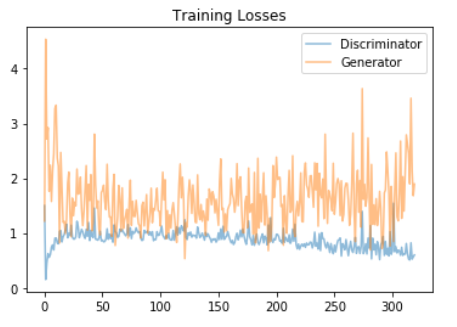The high fluctuation in the Generator training loss is because the input to the Generator Network is a batch of random noise vectors (each of z_size), each sampled from a uniform distribution of (-1,1) to generate new images for each epoch.

In the discriminator plot, we can observe a rise in the training loss (around 50 on the x-axis) followed by a gradual decrease till the end, this is because the Generator has started to generate some realistic image that fooled the Discriminator, leading to increase in error. But slowly as the training progresses, Discriminator becomes better at classifying fake and real images, leading to a gradual decrease in training error.

• Generated samples after 40 epochs.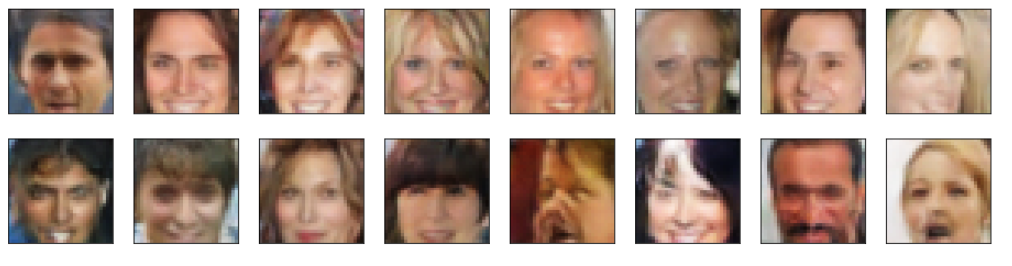Our model was able to generate new images of fake human faces that look as realistic as possible. We can also observe that all images are lighter in shade, even the brown faces are bit lighter. This is because the CelebA dataset is biased; it consists of “celebrity” faces that are mostly white. That being said, DCGAN successfully generates near-real images from mere noise.## Difference between Machine Learning, Data Science, AI, Deep Learning, and Statistics

In this article, I clarify the various roles of the data scientist, and how data science compares and overlaps with related fields such as machine learning, deep learning, AI, statistics, IoT, operations research, and applied mathematics.

## Most popular Data Science and Machine Learning courses — July 2020

Most popular Data Science and Machine Learning courses — August 2020. This list was last updated in August 2020 — and will be updated regularly so as to keep it relevant

## Cheat Sheets for AI, Neural Networks, Machine Learning, Deep Learning & Big Data

Cheat Sheets for AI, Neural Networks, Machine Learning, Deep Learning & Big Data

## PyTorch for Deep Learning | Data Science | Machine Learning | Python

PyTorch for Deep Learning | Data Science | Machine Learning | Python. PyTorch is a library in Python which provides tools to build deep learning models. What python does for programming PyTorch does for deep learning. Python is a very flexible language for programming and just like python, the PyTorch library provides flexible tools for deep learning.

## Data Augmentation in Deep Learning | Data Science | Machine Learning

Data Augmentation is a technique in Deep Learning which helps in adding value to our base dataset by adding the gathered information from various sources to improve the quality of data of an organisation.